# The basic laws of pneumatics

Without pressure nothing works!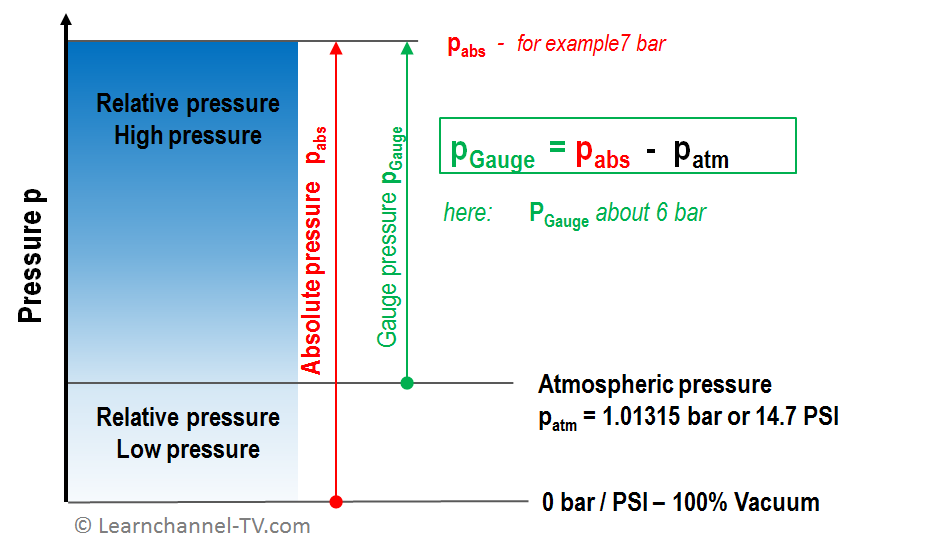Pressure in Pneumatics - Definitions

Patm  atmospheric pressure, that means the pressure at sea level
PGauge     atmospheric differential pressure
Pabs   Pressure in absolute numbers in relation to 0 bar or vacuum. (abb. from pressure absolute)

Units:

Pascal (Pa) represents the pressure unit of the International System of Units (SI), but in industrial practice, pressure is represented in bar or pounds per square inch (PSI).
1 bar = 105 Pa =14.5 PSI

1 Pascal=1 Newton
1 m2

1 PSI=1 pound
1 in 2

#### The law of physics according to Boyle-Mariotte (Robert Boyle e Edna Mariotte)

The air, which is condensed has the effort to generate pressure. In other words, the bigger the compression, the bigger the pressure (assuming temperature remains constant):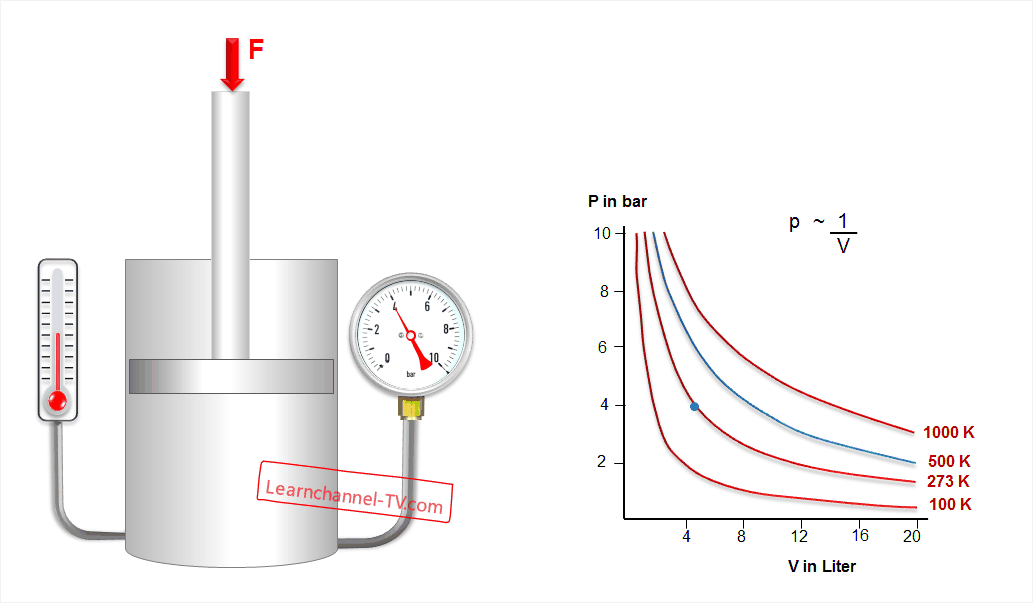Law of Boyle-Mariotte

p ~ 1 / V at T = constant (Isothermal curve): Each curve belongs to a certain temperature and is therefore called isotherm. The curves form a hyperbola. You can explain this behaviour by regarding the air particles as molecules, which bump against the vessel wall. If the volume is reduced, these particles are given less space. So they will strike more often against the vessel wall and thereby the mean force increases.

#### Law  of Gay Lussac (Joseph Louis gay Lussac)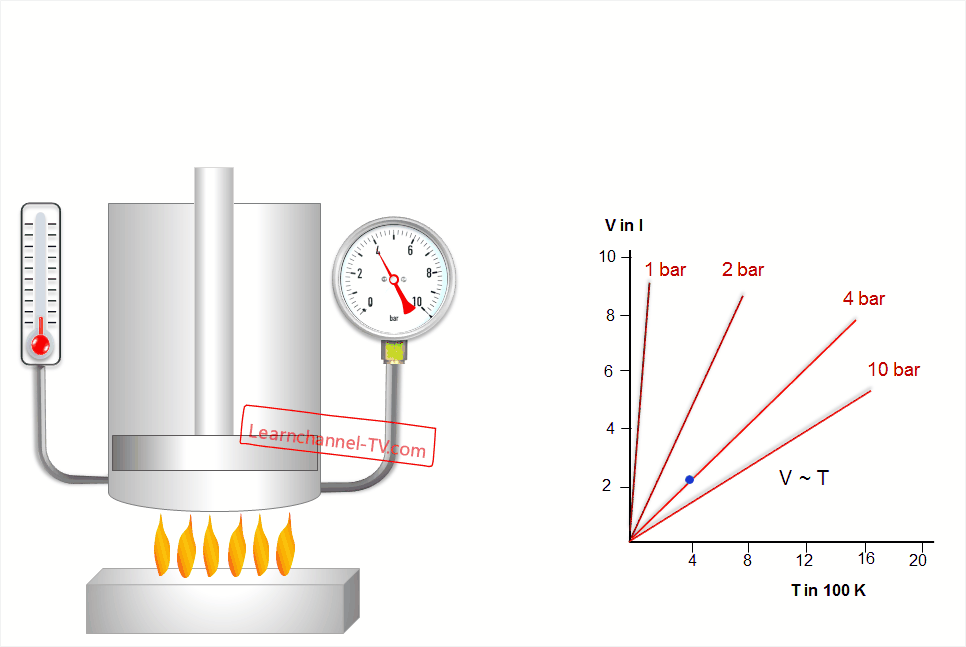Law of Lussac

V ~ T at p = const. (Isobar change): If the pressure p is to be kept constant, then the particles have to be given larger volumes at higher temperatures. The straight lines (V ~ T) are called isobars.

#### Law of Charles (Jacques Alexandre Charles)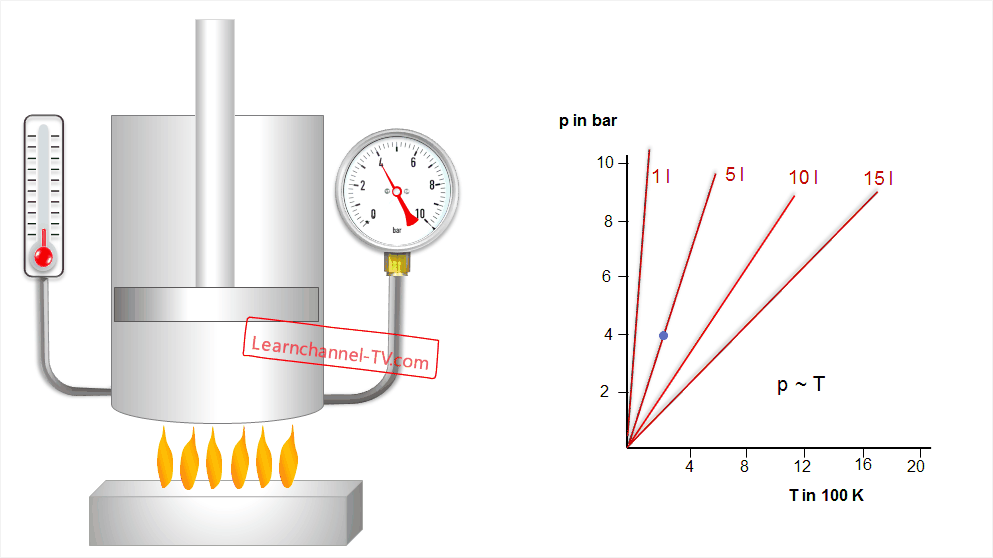Law of Charles

p ~ T at V = const. (Isochronic curve): The explanation is simple: the higher the temperature, the higher the velocity of the particles, and the more often these particles strike the vessel wall. The lines (p ~ T) are called isochors.

General gas equation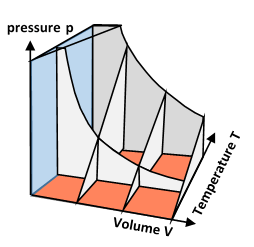Pneumatics - General gas equation

Gas has the property of expanding when the temperature increases. The general gas equation takes into account the influence of temperature on a closed gas quantity.

p1 * V1  =  p2 * V2
T1            T2

.

.

.

Exercises for chapter  2:

2A1: For a plant, a working pressure of 6 bar refering to the norm-pressure of 1.01325 bar is to be generated. What must be Pabs?
2A2: A compressor compresses the ambient air to 1/8 of its volume. What pressure will be achieved by the simplification that the temperature remains constant?